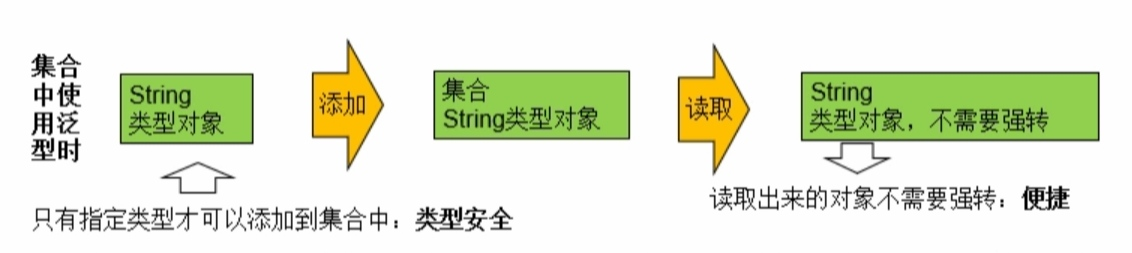## Java学习day32-泛型

1.泛型，JDK1.5新加入的，解决数据类型的安全问题，其主要原因是在类声明时通过一个标示表示类中某个属性的类型或者是某个方法的返回值及参数类型。这样在类声明或实例化时只要指定好需要的具体的类型即可。

2.Java泛型可以保证如果程序在编译时没有发出警告，运行时就不会产生ClassCastException异常。同时，代码更加简洁、健壮。

3.Java中的泛型，只在编译阶段有效。在编译过程中，正确检验泛型结果后，会将泛型的相关信息擦除，并且在对象进入和离开方法的边界处添加类型检查和类型转换的方法。也就是说，泛型信息不会进入到运行阶段。```package day13;

import java.util.ArrayList;
import java.util.List;

public class Test {
public static void main(String[] args) {
List list = new ArrayList();
System.out.println(list);

//需求，只能在集合中存字符串
List<String> l = new ArrayList<String>();
System.out.println(l);

}
}```

二、泛型的使用

1.泛型类

①对象实例化时不指定泛型，默认为：Object。

②泛型不同的引用不能相互赋值。

```public class Test {
public static void main(String[] args) {

A<String> a1 = new A<String>();//在new A的对象指定泛型的类型String
a1.setKey("xxx");//对象使用serKey(T key)方法中的key形参就是String类型
String s = a1.getKey();//T getKey()，返回值就有new对象确定返回值是String

A<Integer> a2 = new A<Integer>();
a2.setKey(1);
a2.getKey();

A a3 = new A();//不指定泛型，相当于指定了一个Object类型
//等同于A<Object> a3 = new A<Object>();
a3.setKey(new Object());
Object obj = a3.getKey();

//同样的类，但是在new对象时泛型指定不同的数据类型，这些对象不能互相赋值。
}
}

/**
* 此处的泛型T可以任意取名
* 一般使用大写的T，意为Type
* */
class A<T>{
private T key;

public void setKey(T key){
this.key = key;
}

public T getKey(){
return this.key;
}
}```

2.泛型接口

```//定义一个泛型接口
interface Generator<T>{
T next();
}```
```package day13;

public class Text1 {
public static void main(String[] args){
B1<Object> b1 = new B1<Object>();
B1<String> b2 = new B1<String>();

B2 b3 = new B2();
}
}

interface IB<T>{
T test(T t);
}

/**
* 未传入泛型实参时，与泛型类的定义相同，在声明类的时候，需将泛型的声明也一起加到类中
* */
class B1<T> implements IB<T>{

public T test(T t) {
// TODO Auto-generated method stub
return t;
}
}

/**
* 如果实现接口时指定接口的泛型的具体数据类型
* 这个类实现接口的所有方法的位置都要泛型替换实际的具体的数据类型
* */
class B2<String> implements IB<String>{

public String test(String t) {
// TODO Auto-generated method stub
return null;
}

}```

3.泛型方法

①方法，也可以被泛型化，不管此时定义在其中的类是不是泛型化的。在泛型方法中可以定义泛型参数，此时，参数的类型就是传入数据的类型。

②泛型方法的格式：

```public class DAO{
public <E> void show(E e){
System.out.println(e.toString());
}
public <T> T show1(T t){
return t;
}
}```
```package day13;

public class Text1 {
public static void main(String[] args){

Cc<Object> c = new Cc<Object>();
c.test("xxx");
//泛型方法在调用之前没有固定的数据类型
//在调用时，传入的参数是什么类型，就会把泛型改成什么类型
//也就是说泛型方法会在调用时确定泛型的具体数据类型
Integer i = c.test1(2);//传递的参数是Integer，泛型就固定成Integer，返回值就是Integer。
Boolean b= c.test1(true);//传递的参数是Boolean，泛型就固定成Boolean，返回值就是Boolean。
}
}

class Cc<E>{
private E e;

/**
* 静态方法的泛型方法
* */
public static <T> void test3(T t){
//在静态的方法中，不能使用类定义的泛型，如果要使用泛型，只能使用静态方法自己定义的泛型
//        System.out.println(this。e);
System.out.println(t);
}

/**
* 无返回值的泛型方法
* */
public <T> void test(T s){
System.out.println(this.e);
//在类上定义的泛型，可以在普通的方法中使用
T t = s;
}

/**
* 有返回值的泛型方法
* */
public <T> T test1(T s){
return s;
}

/**
* 形参为可变参数的泛型方法
* */
public <T> void test2(T... strs){
for(T s : strs){
System.out.println(s);
}
}
}```

4.通配符

```/**
*不确定集合中的元素具体的数据类型
*使用？表示所有类型
*/
public void test(List<?> list){
System.out.println(list);
}```

①有限制的通配符

```//举例
<? extends Person>   (无穷小, Person]

<? super Person>   (Person, 无穷大]

<? super Comparable>

```package day13;

import java.util.ArrayList;
import java.util.List;

public class Test2 {
public static void main(String[] args){
Dd d = new Dd();
List<String> l1 = new ArrayList<String>();
d.test(l1);

List<Integer> l2 = new ArrayList<Integer>();
d.test(l2);

List<C1> lc = new ArrayList<C1>();
d.test1(lc);

List<D1> ld = new ArrayList<D1>();
d.test1(ld);

//        List<B1> lb = new ArrayList<B1>();
//        d.test1(lb);//错误，test1的范围是C1及其子类，B1是C1父类

d.test2(lc);
List<A1> la = new ArrayList<A1>();
d.test2(la);
//        d.test2(ld);//错误，test2的范围是C1及其f父类，D1是C1子类

List<IAImpl> lia = new ArrayList<IAImpl>();
d.test3(lia);

//        d.test3(la);//错误，la不是A1的实现类
}
}

class Dd{
public void test(List<?> list){//假设test方法需要一个list集合的参数，不确定list集合到底存的数据类型是什么

}

public void test1(List<? extends C1> list){//list参数的元素数据类型是C1及其子类

}

public void test2(List<? super C1> list){//list参数的元素数据类型是C1及其父类

}

public void test3(List<? extends IA> list){//list参数的元素数据类型是IA的实现类

}
}

class A1{}

class B1 extends A1{}

class C1 extends B1{}

class D1 extends C1{}

interface IA{}

class IAImpl implements IA{}```